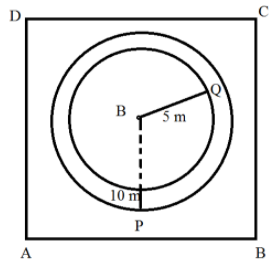QuestionAnswers

# A horse is tied to a peg at one corner of a square shaped grass field of side $15m$ by means of a $5m$ long rope. Find (i)the area of that part of that part of the field in which the horse can graze(ii)The increase in the grazing area if the rope were 10 m long instead of 5m (Use =3.14)Verified
128.7k+ views
Hint: Let ABCD be square filed and the length the of rope $= 5m(r)$ we need to find Area of the field which horse can graze i.e. Area of sector QBP.
In square all angles are $90$ Hence$\angle QBP = 90$
In II nd part length of rope is increased to $10m$
Area gazed by horse now $=$ Area of sector HBG
And increasing in grazing Area$=$Area grazed by horse Now-Area grazed previously

Complete step-by- step solution:Let ABCD be square filed and the length of rope $= 5m(r)$ here the area of the field which a horse can graze is nothing but the Area of sector QBP.

(i)Here we have to find the part of that part of the field in which the horse can graze
Area of sector $= \dfrac{\theta }{{360}} \times \pi {r^2}$
We can call it sector in which horse graze the area
Where r=length of rope
$\theta = {90^0},\pi = \dfrac{{22}}{7}$
On substituting the value in $eq(i)$ we get
Area of sector $= \dfrac{{90}}{{360}} \times \dfrac{{22}}{7} \times {5^2}$
$= 19.625{m^2}$
(ii)If the rope is increased by the $10m$ long the the area of the area gazed by horse now $=$ Area of sector HBG
So, new area $= \dfrac{{90}}{{360}} \times \dfrac{{22}}{7} \times {(10)^2}$
$= 78.5{m^2}$
We have to find increased in area which is nothing but subtracting the old area from new area
Increased area $=$New area-old area
Increased area$= 78.5 - 19.625$
Increased area$= 58.875{m^2}$

Note: The area available for here to graze us nothing but “area of quadrant of a circle”
The axes of a two-dimensional Cartesian system divide the plane into four infinite regions, called quadrants, each bounded by the two half-axes. Quadrant consists of four coordinates.
$= \dfrac{{\pi \times {r^2}}}{4}$
$= \dfrac{{3.14 \times 5 \times 5}}{4}$
$= 19.625{m^2}$------->(old area)
Area$= 78.5{m^2}$------>(new area)
Increased area $=$New area-old area
Increased area$= 78.5 - 19.625$
Increased area$= 58.875{m^2}$